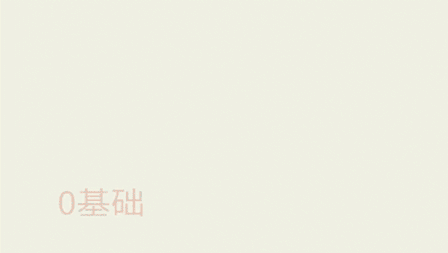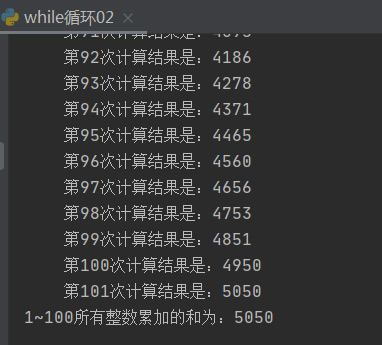# python while循环语句的用法【代码体验】while 语句基本语法

while 条件(判断 计数器 是否达到 目标次数):

条件满足时，做的事情1

条件满足时，做的事情2

条件满足时，做的事情3

...(省略)...

处理条件(计数器 + 1)

# 打印输出5遍“你好！黑马程序员”
i = 1
while i <= 5:
print("你好！黑马程序员")
i += 1

result = 0
i = 0
while i <= 100:
result += i
i += 1
print('\t第%d次计算结果是：%d' % (i, result))
print('1~100所有整数累加的和为：%d' % result)# 求0~100以内偶数的和
result = 0
i = 0
while i <= 100:
if i % 2 == 0:
result += i
print('这次偶数为：%d ，相加结果为：%d' % (i, result))
i += 1
print(result)(1) break

·在循环过程中，如果某一个条件满足后，不再希望循环继续执行，可以使用 break 退出循环

(2) continue

while循环嵌套

while 条件 1:
条件满足时，做的事情1
条件满足时，做的事情2
条件满足时，做的事情3
...(省略)...
while 条件 2:
条件满足时，做的事情1
条件满足时，做的事情2
条件满足时，做的事情3
...(省略)...
处理条件 2
处理条件 1

row = 1
while row <= 9:
column = 1
while column <= row:
result = row * column
# print默认end属性为“\n”，需要去掉改属性
print("%d * %d = %d\t" % (column, row, result), end="")
column += 1
# 结束end=''的影响，另起一行
print("")
row += 1Python入门到精通视频教程下载

JavaEE